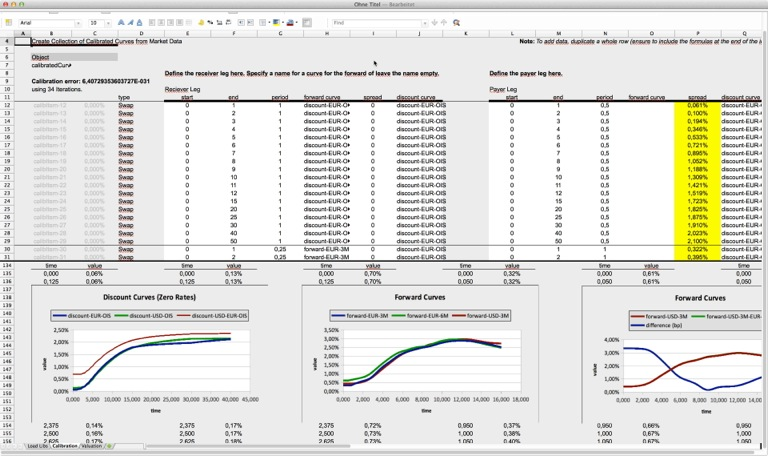finMath.net

# Curve Calibration

## Methodology

For details on the methodology see
Fries, Christian P.: Curves: A Primer. Definition, Calibration and Application of Rate Curves. (December 27, 2012). http://ssrn.com/abstract=2194907.

### Implementation

The calibration of the model is performed as a multi-threaded global optimization. Hence the calibration greatly profits from a multi-core architecture. It uses as many threads as degree of freedoms (e.g. calibrating 5 curves, each with 20 points will use up to 100 threads).

You may explore the algorithm via a spreadsheet (user perspective) or programmatically by checking out the source code (developer perspective).Spreadsheet interface to Java class CalibratedCurves.

The spreadsheets are given in Excel (xls) and OpenOffice (ods) format.

In order to run the spreadsheet you have to install the Java Object Handler for Spreadsheets, "Obba".

Curve Calibration.zip
Calibration of curves (see package net.finmath.marketdata.model.curves).

The sheet calibrates a set of different curves (including discounting curves (e.g., OIS) and forward curves) from swaps. Swaps may feature different discounting curves (e.g., OIS discounting). Forward curves can be calibrated to standard swaps and tenor basis swaps. Discount curves may be calibrated to standard swaps or cross-currency basis swaps.

Although the specific algorithm used is a calibration and not a classical bootstrap, this is sometimes called as curve bootstrapping.

## Source Code

Source code is available from the finmath lib repository, see http://www.finmath.net/finmath-lib.

Below you find a short description about the classes involved in the calibration algorithm. If you like to explore the source code:

• Checkout finmath lib (for example via Eclipse and the Git repository).
• Run the class the class CalibrationTest from the package net.finmath.tests.marketdata.curves.
• Inspect that class.

The calibration framework consists of three parts:

• The implementation of curves (discount curve, forward curve).
• The implementation of calibration products (swap leg, swap).
• The implementation of a solver, wrapping curves into an parameter object and wrapping calibration products into an objective function object.

### Curves

The curves provide methodology for creating a interpolating curve from a set of points via different interpolation methods on different interpolation entities.

A forward curve can interpolate on the forward value by using its associated discount curve.

Curve are aggregated in an analytic model, which is a collection of curves (Map<String,Curve>) which can be used to evaluate the products.### Calibration Products

Products are object carrying the property of the product, including the name of the curves they reference. They provide a function to taking an analytic model (mapping curve names to Curve objects) which returns the value of the product.### Calibration (Solver)

The object CalibratedCurves takes a set of calibration specifications and creates all the required curves and calibration products. The result of this process is

• A model, basically a collection of curves, used to value the calibration products.
• A collection of curves to calibrate, this may be a smaller subset of the model.
• A collection of calibration products.
It performs of the calibration products finding the best curves for the set of curves to calibrate.

Products are valued using an AnalyticModel, which is just a collection of curves (Map<String,CurveInterface>).

The actual optimization is performed by the class Solver. This class returns a modified clone of the provided model containing calibrated version of curves (the original model and the original curves are not modified).